# Integer

The integers (Latin word integer means "untouched") are the natural numbers (1, 2, 3, …), their negatives (−1, −2, −3, ...) and zero. They are also called whole numbers.

More formally, the integers are the only integral domain whose positive elements are well-ordered, and this order is preserved by addition. Like the natural numbers, the integers form a countably infinite set. The set of all integers is usually denoted by a boldface Z (or blackboard bold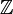$\mathbb{Z}$, or Unicode ℤ), which stands for Zahlen (German for "numbers").

The term rational integer is used in algebraic number theory to distinguish these "ordinary" integers, embedded in the field of rational numbers, from other "integers" such as the algebraic integers.

## Algebraic properties

Like the natural numbers, Z is closed below the operations of addition and multiplication, that is, the sum and product of any two integers is an integer. Also, unlike the natural numbers, it is closed under subtraction. Z is not closed under the operation of division, since the quotient of two integers (e.g., 1 divided by 2), need not be an integer.

The following lists some of the basic properties of addition and multiplication for any integers a, b and c.

 addition multiplication closure: a + b   is an integer a × b   is an integer associativity: a + (b + c)  =  (a + b) + c a × (b × c)  =  (a × b) × c commutativity: a + b  =  b + a a × b  =  b × a existence of an identity element: a + 0  =  a a × 1  =  a existence of inverse elements: a + (−a)  =  0 distributivity: a × (b + c)  =  (a × b) + (a × c) No zero divisors: if ab = 0, then either a = 0 or b = 0 (or both)

In the language of abstract algebra, the first five properties listed above for addition say that Z under addition is an abelian group. As a group under addition, Z is a cyclic group, since every nonzero integer can be written as a finite sum 1 + 1 + ... 1 or (−1) + (−1) + ... + (−1). In fact, Z under addition is the only infinite cyclic group, in the sense that any infinite cyclic group is isomorphic to Z.

The first four properties listed above for multiplication say that Z under multiplication is a commutative monoid. However, note that not every integer has a multiplicative inverse; e.g. there is no integer x such that 2x = 1, because the left hand side is even, while the right hand side is odd. This means that Z under multiplication is not a group.

All the properties from the above table, except for the last, taken together say that Z together with addition and multiplication is a commutative ring with unity. Adding the last property says that Z is an integral domain. In fact, Z provides the motivation for defining such a structure.

The lack of multiplicative inverses, which is equivalent to the fact that Z is not closed under division, means that Z is not a field. The smallest field containing the integers is the field of rational numbers. This process can be mimicked to form the field of fractions of any integral domain.

Although ordinary division is not defined on Z, it does possess an important property: given two integers a and b with b ≠ 0, there exist unique integers q and r such that a = q × b + r and 0 ≤ r < |b|, where |b| denotes the absolute value of b. The integer q is called the quotient and r is called the remainder, resulting from division of a by b. This is the basis for the Euclidean algorithm for computing greatest common divisors.

Again, in the language of abstract algebra, the above says that Z is a Euclidean domain. This implies that Z is a principal ideal domain and any positive integer can be written as the products of primes in an essentially unique way. This is the fundamental theorem of arithmetic.

## Types of integers

• Positive integers is a set ℤ+ ∈ ℤ which contains only positive numbers (same as natural numbers);
• Negative integers is a set ℤ- ∈ ℤ which contains only negative numbers;
• Non-positive integers is a subset of ℤ which contains negative integers and zero (ℤ- ∪ {0});
• Non-negative integers is a subset of ℤ which contains positive integers and zero (ℤ+ ∪ {0});
• Almost integer is a number that is very close to an integer;
• Radical integers is a set of numbers, that is closed under addition, multiplication, subtraction and root extraction.

## Order-theoretic properties

Z is a totally ordered set without upper or lower bound. The ordering of Z is given by

... < −2 < −1 < 0 < 1 < 2 < ...

An integer is positive if it is greater than zero and negative if it is less than zero. Zero is defined as neither negative nor positive.

The ordering of integers is compatible with the algebraic operations in the following way:

1. if a < b and c < d, then a + c < b + d
2. if a < b and 0 < c, then ac < bc. (From this fact, one can show that if c < 0, then ac > bc.)

It follows that Z together with the above ordering is an ordered ring.

## Conversion of real numbers to integers

For more information, see: Floor or ceiling functions.

It is possible to convert a real number to an integer. One way is called rounding, when a real number is rounded down if its fractional part less than 5, and up if more than or equal to 5. Also there are two functions called floor and ceiling functions. A floor function of x (denoted as ⌊x⌋) has a value of the biggest number not more than x: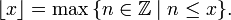$\lfloor x \rfloor=\max\, \{n\in\mathbb{Z}\mid n\le x\}.$

A ceiling function of x (denoted as ⌈x⌉) has a value of the smallest number not less than x: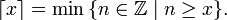$\lceil x \rceil=\min\,\{n\in\mathbb{Z}\mid n\ge x\}.$

Unicode entities for floor function symbols are &#8970 and &#8971, for ceiling function are &#8968 and &#8969.

## Construction

The integers can be constructed from the set of natural numbers by defining them to be the set of equivalence classes of pairs of natural numbers$\mathbb{N} \times \mathbb{N}$ under the equivalence relation

(a,b)∼(c,d) if$a+d = b+c.\,$

Taking 0 to be a natural number, the natural numbers may be considered to be integers by the embedding that maps$n\,$ to$[(n, 0)],\,$ where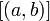$[(a,b)]\,$ denotes the equivalence class having$(a, b)\,$ as a member.

Addition and multiplication of integers are defined as follows:$[(a,b)]+[(c,d)] := [(a+c,b+d)].\,$$[(a,b)]\cdot[(c,d)] := [(ac+bd,ad+bc)].\,$

It is easily verified that the result is independent of the choice of representatives of the equivalence classes.

Typically,$[(a,b)]\,$ is denoted by$\begin{cases} n, & \mbox{if } a \ge b \\ -n, & \mbox{if } a < b, \end{cases}$

where$n = |a-b|.\,$

If the natural numbers are identified with the corresponding integers (using the embedding mentioned above), this convention creates no ambiguity.

This notation recovers the familiar representation of the integers as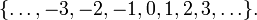$\{\dots, -3, -2, -1, 0, 1, 2, 3, \dots\}.$

Some examples are: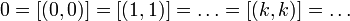$0 = [(0,0)] = [(1,1)] = \dots = [(k,k)] = \dots$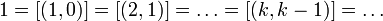$1 = [(1,0)] = [(2,1)] = \dots = [(k,k-1)] = \dots$$-\!$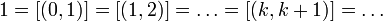$1 = [(0,1)] = [(1,2)] = \dots = [(k,k+1)] = \dots$$2 = [(2,0)] = [(3,1)] = \dots = [(k,k-2)] = \dots$$-\!$$2 = [(0,2)] = [(1,3)] = \dots = [(k,k+2)] = \dots$

## Integers in computing

An integer (sometimes known as an "int", from the name of a datatype in the C programming language) is often a primitive datatype in computer languages. However, integer datatypes can only represent a subset of all integers, since practical computers are of finite capacity. Also, in the common two's complement representation, the inherent definition of sign (mathematics) distinguishes between "negative" and "non-negative" rather than "negative, positive, and 0". (It is, however, certainly possible for a computer to determine whether an integer value is truly positive.)

Variable-length representations of integers, such as bignums, can store any integer that fits in the computer's memory. Other integer datatypes are implemented with a fixed size, usually a number of bits which is a power of 2 (4, 8, 16, etc.) or a memorable number of decimal digits (e.g., 9 or 10).

In contrast, theoretical models of digital computers, such as Turing machines, typically do not have infinite (but only unbounded finite) capacity.